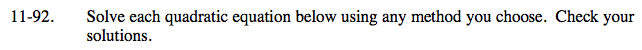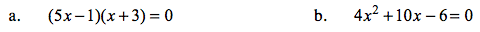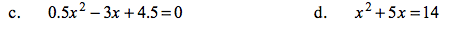### Home > CAAC > Chapter 11 > Lesson 11.2.2 > Problem11-92

11-92.Since its already factored, use the Zero Product Property to solve.

$x=\frac{1}{5}\text{ or }-3$

Factor out a 2 from the equation, then factor the quadratic.

Once factored, see part (a).Multiply throughout by 2 to remove the decimals.

Factor and use the Zero Product Property to solve.
(x + 3)(x + 3) = 0
x = −3

Subtract 14 from both sides, then factor.

Once factored, see parts (a) and (c) for more help.

x = 2 or −7